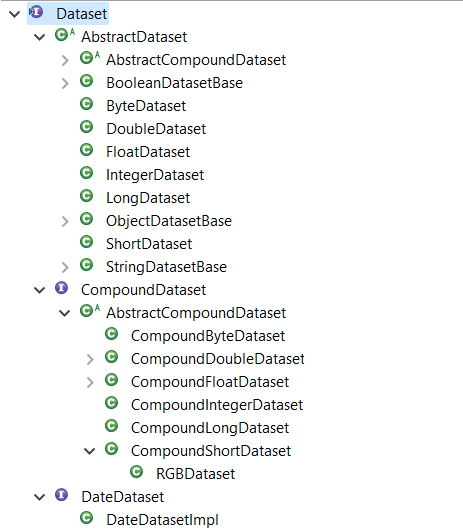# Multi-Dimensional Arrays in Java with Eclipse January

Eclipse January is a set of common data structures in Java, including a powerful library for handling numerical data. As the volumes and complexity of data dramatically increases - the so-called 'Big Data' - Eclipse January provides a numerical library that simplifies the handling and manipulation of data in the form of multi-dimensional arrays.

At the heart of the library are the IDataset and Dataset interfaces and classes. So, here are some reasons you might want to use Eclipse January

• Familiar. Provide familiar functionality, especially to NumPy users.
• Robust. Has test suite and is used in production heavily at Diamond Light Source.
• No more passing double[]. IDataset provide a consistent object for basing APIs on with significantly improved clarity over using double arrays or similar.
• Optimized. Optimized for speed and getting better all the time.
• Scalable. Allows handling of data sets larger than available memory with "Lazy Datasets".
• Focus on your algorithms. By reusing this library it allows you to focus on your code.

This article gives an overview of the functionality of the Dataset class for multi-dimensional arrays.

## Array Creation

Eclipse January supports straightforward creation of arrays. Let's say we want to create a 2-dimensional array with the following data:

```[1, 2, 3,
4, 5, 6,
7, 8, 9]```

First we can create a new dataset:

``` Dataset dataset = DatasetFactory.createFromObject(new double[] { 1, 2, 3, 4, 5, 6, 7, 8, 9 }); ```

This gives us a 1-dimensional array with 9 elements, as shown below:

```[1.0000000, 2.0000000, 3.0000000, 4.0000000, 5.0000000, 6.0000000, 7.0000000, 8.0000000, 9.0000000]
```

We then need to reshape it to be a 3x3 array:

``` dataset = dataset.reshape(3, 3); ```

The reshaped dataset:

``` [[1.0000000, 2.0000000, 3.0000000],
[4.0000000, 5.0000000, 6.0000000],
[7.0000000, 8.0000000, 9.0000000]]
```

Or we can do it all in just one step:

``` Dataset another = DatasetFactory.createFromObject(new double[] { 1, 1, 2, 3, 5, 8, 13, 21, 34 }).reshape(3, 3); ```

Another dataset:

``` [[1.0000000, 1.0000000, 2.0000000],
[3.0000000, 5.0000000, 8.0000000],
[13.000000, 21.000000, 34.000000]]
```

There are methods for obtaining the shape and number of dimensions of datasets

``` System.out.println("shape of dataset: " + Arrays.toString(dataset.getShape())); System.out.println("number of dimensions: " + dataset.getRank()); ```

Which gives us:

```shape of dataset: [3, 3]
number of dimensions: 2
```

Datasets also provide functionality for ranges and a random function that all allow easy creation of arrays:

``` Dataset a = DatasetFactory.createRange(15, Dataset.INT32).reshape(3, 5); ```
```[[0, 1, 2, 3, 4],
[5, 6, 7, 8, 9],
[10, 11, 12, 13, 14]]
```
``` Dataset rand = Random.rand(new int[]{3,4});```
```[[0.37239989, 0.89414117, 0.94036325, 0.47739019],
[0.47194246, 0.12534931, 0.41001452, 0.90583666],
[0.81731075, 0.76468139, 0.97097539, 0.37182491]]
```

IDataset is not just for doubles, it can also be used with other types such as:

• int, float
• complex
• Compound types such as RGB
• String
• Any class really!Figure 1: DataSet Type Hierarchy

## Array Operations

The org.eclipse.january.dataset.Maths provides rich functionality for operating on the Dataset classes. For instance, here's how you could add 2 Dataset arrays:

``` Dataset add = Maths.add(dataset, another); ```

Or you could do it as an inplace addition. The example below creates a new 3x3 array and then adds 100 to each element of the array.

``` Dataset inplace = DatasetFactory.createFromObject(new double[] { 1, 2, 3, 4, 5, 6, 7, 8, 9 }).reshape(3, 3); inplace.iadd(100); ```
```[[101.0000000, 102.0000000, 103.0000000],
[104.0000000, 105.0000000, 106.0000000],
[107.0000000, 108.0000000, 109.0000000]]```

The Math library also provides functionality for:

• Exponential & logarithmic operations
• Stats: min, max, mean, median, quantiles, covariance, kurtosis, etc
• Trigonometric Functions: sin, cos, tan, etc
• And more!

Additionally there is also a LinearAlgebra class that operates on Datasets.

## Slicing

Datasets simplify extracting portions of the data, known as 'slices'. For instance, given the array below, let's say we want to extract the data 2, 3, 5 and 6.

```[1, 2, 3,
4, 5, 6,
7, 8, 9]
```

This data resides in the first and second rows and the second and third columns. For slicing, indices for rows and columns are zero-based. A basic slice consists of a start and stop index, where the start index is inclusive and the stop index is exclusive. An optional increment may also be specified. So this example would be expressed as:

``` Dataset slice = dataset.getSlice(new Slice(0, 2), new Slice(1, 3)); ```

slice of dataset:

```[[2.0000000, 3.0000000],
[5.0000000, 6.0000000]]
```

Slicing and array manipulation functionality is particularly valuable when dealing with 3-dimensional or n-dimensional data.

### Try Eclipse January

The Getting Started Guide shows how you can get started with the example project in Eclipse. Most of the code in this article is from the BasicExample class. Once you've tried the basics, there are some more advanced examples to have a look at and run:

• NumPy Examples shows how common NumPy constructs map to Eclipse Datasets.
• Slicing Examples demonstrates slicing, including how to slice a small amount of data out of a dataset too large to fit in memory all at once.
• Error Examples demonstrates applying an error to datasets.
• Iteration Examples demonstrates a few ways to iterate through your datasets.
• Lazy Examples demonstrates how to use datasets which are not entirely loaded in memory.

Eclipse January is an incubating project from the Science Working Group at Eclipse Foundation. The data structures were developed and used over the years at Diamond Light Source and Oakridge National Labs, two facilities that have a lot of experience dealing with huge, complex amounts of data, in 2-D, 3-D and multi-dimensional formats.

The power of Eclipse January comes not just from the simplicity and convenience of being able to manipulate data, but also provides a basic standard for data storage. This allows for easy integration of tooling based on the Dataset class. So as data sizes continue to grow and be more complex, Eclipse January provides a convenient, powerful, robust library to simplify and standardise multi-dimensional arrays in Java.